Latest Banking jobs   »   Reasoning Ability Quiz For SBI Clerk...

# Reasoning Ability Quiz For SBI Clerk Prelims 2021- 29th May

Directions (1-5): Study the information carefully and answer the questions given below.
An uncertain number of persons sitting in a straight line facing north. Three persons sit between P and L. M sits second to the right of P. Only three persons sit between L and R. S is immediate left of P. M sits fifth from one of the extreme ends of the row. As many persons sit between M and Q as many between Q and R. T sits second to the left of X, who is an immediate neighbor of L. Not more than two persons sit between X and R. Five persons sit between R and Y. As many persons sit between R and Z as many between Z and Y.

Q1. How many persons sitting in a row?
(a) 20
(b) 18
(c) 23
(d) 14
(e) None of these

Q2. Who among the following person sits fourth to the right of Z?
(a) T
(b) X
(c) R
(d) L
(e) None of these

Q3. How many persons are sitting between X and M?
(a) Two
(b) Five
(c) Three
(d) Four
(e) More than five

Q4. If J is an immediate neighbor of S then who among the following person sits second to the left of J?
(a) P
(b) X
(c) L
(d) Q
(e) None of these

Q5. Four of the following five are alike in a certain way based on a group, find the one that does not belong to that group?
(a) R-X
(b) Q-P
(c) M-S
(d) Z-T
(e) L-T

Q6. If each vowel of the word “Quagmire” is changed to the next in the English alphabetical series and each consonant to the previous letter in the English alphabetical series and then the alphabets thus formed are arranged in alphabetical order from left to right, which of the following will be fifth from the left end?
(a) F
(b) J
(c) P
(d) Q
(e) None of these

Q7. If MEDIATION= 945%6#%8\$ and REATTACH = &46##613, then how will you code ANOTHER?
(a) 6\$8#34\$
(b) 6\$8#34&
(c) 4\$8#34\$
(d) 6\$5#34\$
(e) None of these

Q8. In a certain code, AUDIENCE is written as UAIDNEEC. How is ‘ATTORNEY’ coded as?
(a) ATOTNRYE
(b) TAOTNRYE
(c) TAOTNREY
(d) TATONRYE
(e) None of these

Q9. How many pairs of letters are there in the word “CONVERSATION” each of which have as many letters between them in the word as they have between them in the English alphabetical series?
(a) More than four
(b) Three
(c) Four
(d) Two
(e) One

Q10. Which alphabet is 3rd from the left in the meaningful four letter word formed from the 2nd, 4th and 8th, and 11th letter of the word “PRODUCTIVITY”? If more than one word is formed then mark answer as X and no meaningful word is formed then mark answer as Z.
(a) T
(b) Z
(c) R
(d) X
(e) D

Direction (11-12): Study the given information and answer the following question.
Point A is 13m west of point B. Point D is 4m south of point B. Point C is east of 9m of point M. Point T is 12m west of point D. Point Y is 16m north of point C. Point Y is 2m west of point A.

Q11. What is the direction of C with respect of T?
(a) North-west
(b) South
(c) North-east
(d) West
(e) None of these

Q12. In which direction of Point A with respect to Point T?
(a) North
(b) North-east
(c) East
(d) North-west
(e) None of these

Directions (13-15): Study the following information carefully and answer the given questions.
Point S is 40 m towards the North of Point P. Point Q is 15 m towards the West of point S. Point U is 25 m towards the West of point S. Point T is 20 m towards the South of point Q. Point R is 15 m towards the East of point T. Point V is 15 m towards the West of point P.
Q13. Which of the following points are in a straight line?
(a) S, T, P
(b) T, V, R
(c) S, Q, V
(d) T, V, Q
(e) U, Q, R

Q14. P is in which direction with respect to R?
(a) South
(b) North
(c) East
(d) West
(e) Cannot be determined

Q15. If a person walks 10 m towards East from point U and then takes a right turn, which of the following points would he reach first?
(a) V
(b) S
(c) T
(d) P
(e) R

Practice More Questions of Reasoning for Competitive Exams:

###### SBI Clerk Study Plan 2021

Solutions

Solutions (1-5):
Sol.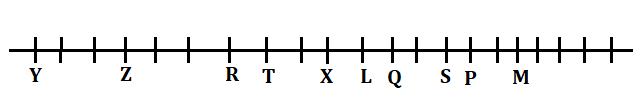S1.Ans (e)
S2.Ans (a)
S3.Ans (e)
S4.Ans (c)
S5.Ans (d)

S6. Ans (e)
Given word- Quagmire
Obtained word- BFFJLPQV

S7. Ans (b)

S8.Ans (b)
Sol.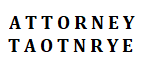S9. Ans. (a)

S10. Ans. (c)
Sol. DIRT

Solutions (11-12):
Sol.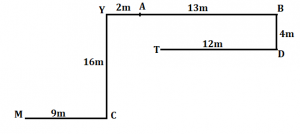S11.Ans (e)
S12.Ans (d)

Solutions (13-15):
Sol.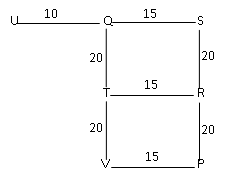S13. Ans.(d)
S14. Ans.(a)
S15. Ans.(c)#### Congratulations!Incorrect details? Fill the form again here

•Reasoning Ability Quiz For IBPS RRB PO C...
•Reasoning Ability Quiz For IBPS Clerk Pr...
•Reasoning Ability Quiz For IBPS RRB PO P...
•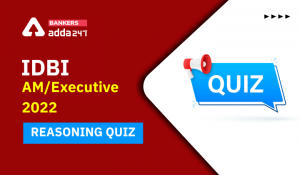Reasoning Ability Quiz For IDBI AM/Execu...
•Reasoning Ability Quiz For IBPS RRB PO P...
•Reasoning Ability Quiz For IDBI AM/Execu...